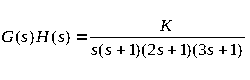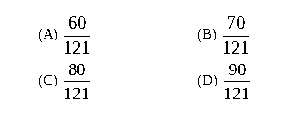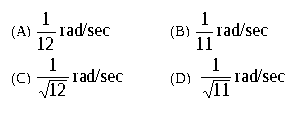# (GATE 2011) Examination Structure

## GATE Examination Structure

A candidate can apply for only ONE of the 21 papers listed in the table given below. In the tables given below, the columns titled 'Paper Title' and 'Section Title' provide links to the appropriate syllabus.

The choice of the appropriate paper is the responsibility of the candidate. Some guidelines in this respect are suggested below.

The candidate is expected to appear in a paper appropriate to the discipline of his/her qualifying degree. The candidate is, however, free to choose any paper according to his/her admission plan, keeping in mind the eligibility criteria of the institutions in which he/she wishes to seek admission.

Requests for change of Test paper after the submission of Application Form will not be considered.

## Patterns of Qustion Papers

Pattern for Papers: AG, AR, BT, CE, CH, CS, CY, EC, EE, IN, MA, ME, MN, MT, PH and PI:

There will be a total of 65 questions carrying 100 marks.

Q.1 to Q.25 (25 questions) carry one mark each (sub-total 25 marks). Q.26 to Q.55 (30 questions) carry two marks each (sub-total 60 marks). Questions Q.56 - Q.65 belong to General Aptitude (GA). Questions Q.56 - Q.60 (5 questions) carry 1 mark each (sub-total 5 marks) and questions Q.61 - Q.65 (5 questions) carry 2-marks each (sub-total 10 marks).

Questions Q.48 - Q.51 (2 pairs) are common data questions. and Qquestion pairs (Q.52, Q.53) and (Q.54, Q.55) are linked answer questions. The answer to the second question of the linked answer questions depends on the answer to the first question of the pair. If the first question in the linked pair is wrongly answered or is unattempted, then the answer to the second question in the pair will not be evaluated.

NEGATIVE MARKING: For Q.1 - Q.25 and Q.56 - Q.60, ⅓ mark will be deducted for each wrong answer. For Q.26 - Q.51 and Q.61 - Q.65, ⅔ mark will be deducted for each wrong answer. The question pairs (Q.52, Q.53), and (Q.54, Q.55) are questions with linked answers. There will be negative marks only for wrong answer to the first question of the linked answer question pair i.e. for Q.52 and Q.54, ⅔ mark will be deducted for each wrong answer. There is no negative marking for Q.53 and Q.55.

Questions on Engineering Mathematics will carry about 15% of the total marks (excluding General Aptitude section) in all the papers bearing the codes AG, BT, CE, CH, CS, EC, EE, IN, ME, MN, MT and PI.

Pattern for Papers: AE and TF:

There will be a total of 65 questions carrying 100 marks.

Q.1 to Q.25 (25 questions) carry one mark each (sub-total 25 marks). Q.26 to Q.55 (30 questions) carry two marks each (sub-total 60 marks). Questions Q.56 - Q.65 belong to General Aptitude (GA). Questions Q.56 - Q.60 (5 questions) carry 1 mark each (sub-total 5 marks) and questions Q.61 - Q.65 (5 questions) carry 2-marks each (sub-total 10 marks).

Questions Q.1-Q.25 and Q.31-Q.65 are of multiple choice type. Questions Q.26-Q.30 are of numerical answer type.

Questions Q.48 - Q.51 (2 pairs) are common data questions. and Qquestion pairs (Q.52, Q.53) and (Q.54, Q.55) are linked answer questions. The answer to the second question of the linked answer questions depends on the answer to the first question of the pair. If the first question in the linked pair is wrongly answered or is unattempted, then the answer to the second question in the pair will not be evaluated.

NEGATIVE MARKING: There is negative marking for multiple choice type questions. For Q.1 - Q.25 and Q.56 - Q.60, ⅓ mark will be deducted for each wrong answer. For Q.31 - Q.51 and Q.61 - Q.65, ⅔ mark will be deducted for each wrong answer. The question pairs (Q.52, Q.53), and (Q.54, Q.55) are questions with linked answers. There will be negative marks only for wrong answer to the first question of the linked answer question pair i.e. for Q.52 and Q.54, ⅔ mark will be deducted for each wrong answer. There is no negative marking for Q.53 and Q.55.

There is no negative marking for numerical answer type questions Q.26-Q.30.

Questions on Engineering Mathematics will carry about 15% of the total marks (excluding General Aptitude section) in all the papers bearing the codes AE and TF

Pattern for Paper: GG:

There are a total of 65 questions carrying 100 marks.

Apart from General Aptitude (GA), the question paper consists of two parts: Part A and Part B. Part A is common for all candidates. Part B contains two sections: Section 1 (Geology) and Section 2 (Geophysics). Candidates will have to attempt questions in Part A and either Section 1 or Section 2 in Part B.

Part A consists of 25 questions; all questions carry 1-mark each (sub-total 25 marks). Each of the sections (Section 1 and Section 2) in Part B consists of 30 questions; all questions carry 2-marks each (sub-total 60 marks). Questions Q.48 - Q.51 (2 pairs) are common data questions. and Qquestion pairs (Q.52, Q.53) and (Q.54, Q.55) are linked answer questions. The answer to the second question of the linked answer questions pair depends on the answer to the first question of the pair. If the first question in the linked pair is wrongly answered or is un-attempted, then the answer to the second question in the pair will not be evaluated. Questions Q.56 - Q.65 belong to General Aptitude (GA). Questions Q.56 - Q.60 (5 questions) will carry 1-mark each (sub-total 5 marks) and questions Q.61 - Q.65 (5 questions) will carry 2-marks each (sub-total 10 marks).

NEGATIVE MARKING: For Q.1 - Q.25 and Q.56 - Q.60, ⅓ mark will be deducted for each wrong answer. For Q.26 - Q.51 and Q.61 - Q.65, ⅔ mark will be deducted for each wrong answer. The question pairs (Q.52, Q.53), and (Q.54, Q.55) are questions with linked answers. There will be negative marks only for wrong answer to the first question of the linked answer question pair i.e. for Q.52 and Q.54, ⅔ mark will be deducted for each wrong answer. There is no negative marking for Q.53 and Q.55.

Pattern for Paper: XE (Engineering Sciences):

There are a total of 65 questions carrying 100 marks.

There are 11 questions carrying 15 marks in XE Engineering Mathematics section paper (Section A), which is compulsory. Questions Q.1 - Q.7 (7 questions) carry 1-mark each (sub-total 7 marks), and questions Q.8 - Q.11 (4 questions) carry 2-marks each (sub-total 8 marks).

Each of the other XE section papers (Sections B through G) contains 22 questions carrying 35 marks. Questions Q.1 - Q.9 (9 questions) carry 1-mark each (sub-total 9 marks). Questions Q.10 - Q.22 (13 questions) carry 2-marks each (sub-total 26 marks). Questions Q.17 - Q.20 (2 pairs) are common data questions with 2-marks each. Questions Q.21 and Q.22 (1 pair) are linked answer questions with 2-marks each. The answer to the second question of the pair of linked answer questions depends on the answer to the first question of the pair. If the first question in the pair is wrongly answered or is unattempted, then the answer to the second question in the pair will not be evaluated.

There are 10 questions carrying 15 marks in General Aptitude (GA), which is compulsory. Questions Q.1 - Q.5 (5 questions) carry 1-mark each (sub-total 5 marks), and questions Q.6 - Q.10 (5 questions) carry 2-marks each (sub-total 10 marks).

Pattern for Paper: XL (Life Sciences):

There are a total of 65 questions carrying 100 marks.

There are 15 questions carrying 25 marks in XL Chemistry section paper (Section H), which is compulsory. Questions Q.1 - Q.5 (5 questions) carry 1-mark each (sub-total 5 marks). Questions Q.6 - Q.15 (10 questions) carry 2-marks each (sub-total 20 marks). Questions Q.12 and Q.13 (1 pair) are common data questions with 2-marks each. Questions Q.14 and Q.15 (1 pair) are linked answer questions with 2-marks each. The answer to the second question of the pair of linked answer questions depends on the answer to the first question of the pair. If the first question in the pair is wrongly answered or is unattempted, then the answer to the second question in the pair will not be evaluated.

Each of the other XL section papers (Sections I through M) contains 20 questions carrying 30 marks. Questions Q.1 - Q.10 (10 questions) carry 1-mark each (sub-total 10 marks) and questions Q.11 - Q.20 (10 questions) carry 2-marks each (sub-total 20 marks).

There are 10 questions carrying 15 marks in General Aptitude (GA), which is compulsory. Questions Q.1 - Q.5 (5 questions) carry 1-mark each (sub-total 5 marks), and questions Q.6 - Q.10 (5 questions) carry 2-marks each (sub-total 10 marks).

## GATE 2011 Question Types

### Multiple Choice Questions

Multiple choice questions in all papers and sections will contain four answers, of which only one is correct. The types of questions in a paper may be based on following logic:

(i) Recall:

These are based on facts, principles, formulae or laws of the discipline. The candidate is expected to be able to obtain the answer either from his/her memory of the subject or at most from a one-line computation.

Example
Q. During machining maximum heat is produced
(A) in flank face (B) in rake face
(C) in shear zone (D) due to friction between chip and tool.

(ii) Comprehension:

These questions will test the candidate's understanding of the basics of his/her field, by requiring him/her to draw simple conclusions from fundamental ideas.

Example
Q. A DC motor requires a starter in order

(A) to develop a starting torque
(B) to compensate for auxiliary field ampere turns
(C) to limit armature current at starting
(D) to provide regenerative braking

(iii) Application:

In these questions, the candidate is expected to apply his/her knowledge either through computation or by logical reasoning.

Example:
Q. The sequent depth ratio of a hydraulic jump in a rectangular channel is 16.48. The Froude number at the beginning of the jump is:

(A) 10.0 (B) 5.0
(C) 12.0 (D) 8.0

(iv) Analysis and Synthesis:

These can be linked questions, where the answer to the first question of the pair is required in order to answer its successor. Or these can be common data questions, in which two questions share the same data but can be solved independently of one another.

### Common data questions

Multiple questions may be linked to a common data problem, passage and the like. Two or three questions can be formed from the given common data problem. Each question is independent and its solution obtainable from the above problem data/passage directly. (Answer of the previous question is not required to solve the next question). Each question under this group will carry two marks.

Example
Common Data, for instance, Questions 48 and 49 in main paper:

Let X and Y be jointly distributed random variables such that the conditional distribution of Y, given X=x, is uniform on the interval (x-1,x+1). Suppose E(X)=1 and Var(X)= 5/3

First question using common data:

Q.48 The mean of the random variable Y is

(A) 1/2 (B) 1 (C) 3/2 (D) 2

Second question using common data:

Q.49 The variance of the random variable Y is

(A) 1/2 (B) 2/3 (C) 1 (D) 2

These questions are of problem solving type. A problem statement is followed by two questions based on the problem statement. The two questions are designed such that the solution to the second question depends upon the answer to the first one. In other words, the first answer is an intermediate step in working out the second answer. Each question in such linked answer questions will carry two marks.

Example:
Statement for Linked Answer Questions, for instance, for Questions 52 and 53 in Main Paper:

The open loop transfer function of a unity feedback control system is given byFirst question of the pair:

Q.52 The value of K which will cause sustained oscillations in the closed loop system isSecond question of the pair:
Q.53 The frequency of sustained oscillations isThe questions based on the above four logics may be a mix of single stand alone statement / phrase / data type questions, combination of option codes type questions or match items types questions.